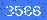位置：首页 > 在线计算器

# 音箱分频器在线计算公式

 C1= μf L1= mH. 相移的一阶分频器是90度

 C1= μf L1= mH C2= μf L2= mHC1=μf L1=mH C2=μf L2=mH L3=mH C3=μfC1=μf C2=μf L2=mH L1=mH C3=μf L3=mH C4=μf L4=mHC1= μf R1= 欧姆L型扬声器喇叭衰减电路

 R1=欧姆 R2=欧姆0/250 验证码：[!--temp.pla--]
栏目ID=10的表不存在(操作类型=0)
www.838dz.com